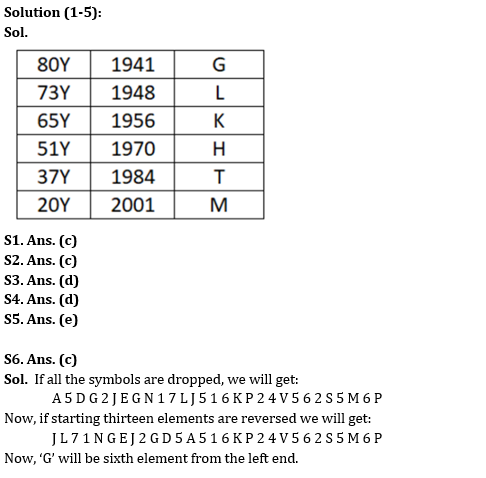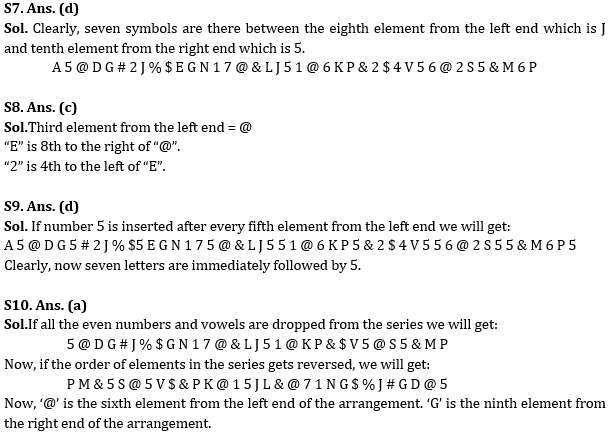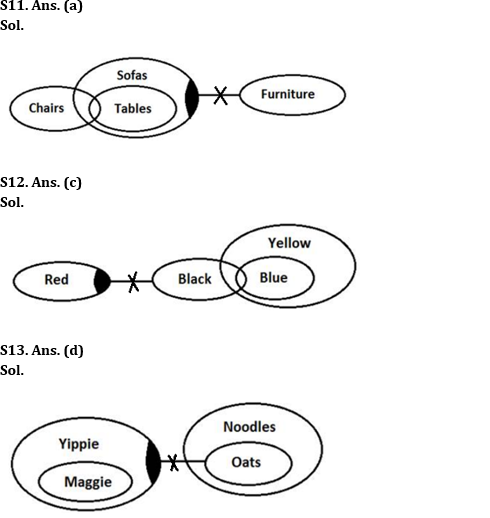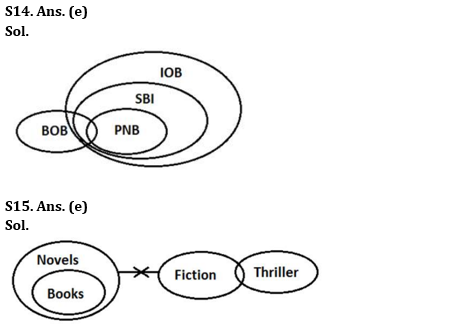Latest Banking jobs   »

# Reasoning Ability Quiz For RBI Grade B/ ECGC PO/ SIDBI Grade A Prelims 2022- 10th May

Directions (1-5): Study the following information carefully and answer the questions accordingly.
Six people are born in six different years i.e. 1941, 1948, 1956, 1970, 1984, and 2001 but not necessarily in the same order. The age is calculated according to the year i.e. 2021. At least two people are older than T. L is older than M but not the oldest. K is born adjacent to H. The age of G is a multiple of 5. At least two people are younger than L. Two people are born between K and M who is not the oldest. Two people are born between H and G.

Q1. Who among the following is the youngest?
(a) L
(b) T
(c) M
(d) H
(e) None of these

Q2. Who among the following is born in 1956?
(a) H
(b) G
(c) K
(d) T
(e) None of these

Q3. What is the sum of the ages of H and G?
(a) 57 years
(b) 153 years
(c) 88 years
(d) 131 years
(e) None of these

Q4. How many letters are there in the English alphabetical series between the youngest and the oldest one?
(a) Three
(b) Eight
(c) Nine
(d) Five
(e) None of these

Q5. Four from the following five are similar in a certain way and thus forms a group. Find out the one, who does not belong to that group.
(a) M
(b) H
(c) G
(d) K
(e) L

Directions (6-10): Study the information given below and answer the questions based on it.

A 5 @ D G # 2 J % \$ E G N 1 7 @ & L J 5 1 @ 6 K P & 2 \$ 4 V 5 6 @ 2 S 5 & M 6 P

Q6. Which of the following element will be sixth from the left end, when all the symbols are dropped and then starting thirteen elements are reversed?
(a) E
(b) N
(c) G
(d) 5
(e) None of these

Q7. How many symbols are there between the eighth element from the left end and tenth element from the right end?
(a) Three
(b) Four
(c) Five
(d) More than five
(e) Two

Q8. Which element is fourth to the left of the element which is eighth to the right of the one which is third from the extreme left end of the series?
(a) #
(b) @
(c) 2
(d) 7
(e) None of these

Q9. In the series, if the number 5 is inserted after every fifth element from the left end then how many pairs are there in the series in which a letter is immediately followed by 5?
(a) Four
(b) Five
(c) Six
(d) More than six
(e) None of these

Q10. If all the even numbers and vowels are dropped from the series and then the order of elements in the series gets reversed, which of the following element is sixth from the left end and ninth from the right end of the arrangement respectively?
(a) ’@’ and ‘G’
(b) ‘G’ and ‘6’
(c) ‘S’ and ‘N’
(d) ‘5’ and ‘\$’
(e) None of these

Directions (11-15): In the question below are given three statements followed by the conclusions. You have to take the given statements to be true even if they seem to be at variance with commonly known facts. Read all the conclusions and then decide which of the given conclusions logically follows from the given statements disregarding commonly known facts.

Q11. Statements: Only a few Chairs are Tables. All Tables are Sofas. Some Sofas are not Furniture.
Conclusions:
I. All Chairs can be Sofas.
II. Some Tables are not Furniture.
(a) Only I follows
(b) Only II follows
(c) Either I or II follows
(d) Neither I nor II follows
(e) Both I and II follow

Q12. Statements: Some Red are not Black. Only a few Black are Blue. All Blue are Yellow.
Conclusions:
I. Some Red are Yellow.
II. No Red are Yellow.
(a) Only I follows
(b) Only II follows
(c) Either I or II follows
(d) Neither I nor II follows
(e) Both I and II follow

Q13. Statements: All Maggie are Yippie. Some Yippie are not Oats. All Oats are Noodles.
Conclusions:
I. No Maggie are Oats.
II. Some Noodles are not Yippie.
(a) Only I follows
(b) Only II follows
(c) Either I or II follows
(d) Neither I nor II follows
(e) Both I and II follow

Q14. Statements: Only a few BOB are PNB. All PNB are SBI. All SBI are IOB.
Conclusions:
I. All PNB are IOB.
II. All BOB can be IOB.
(a) Only I follows
(b) Only II follows
(c) Either I or II follows
(d) Neither I nor II follows
(e) Both I and II follow

Q15. Statements: All Books are Novels. No Novels are Fiction. Only a few Fictions are Thriller.
Conclusions:
I. No Books are Fiction.
II. Some Fictions are not Thriller.
(a) Only I follows
(b) Only II follows
(c) Either I or II follows
(d) Neither I nor II follows
(e) Both I and II follow

Solutions